## SOLVED EXAMPLES

1.  A wave is propagating on a long stretched string along its length taken as the positive x-axis. The wave equation is given as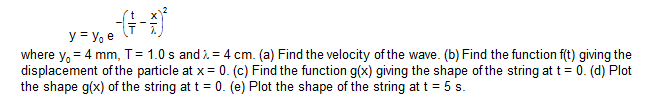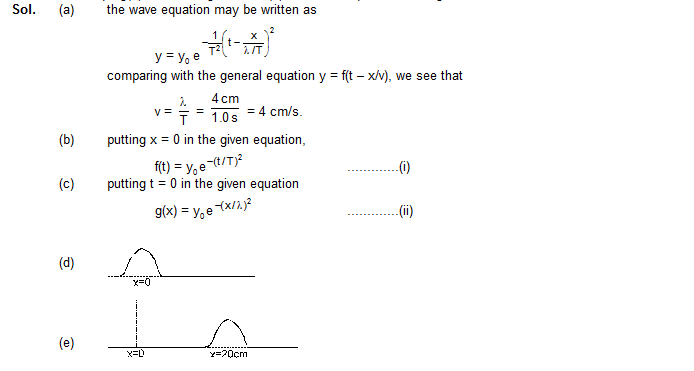2.    Consider the wave y = (5 mm) sin (1 cm–1) x – (60 s–1) t]. Find (a) the amplitude (b) the wave number, (c) the wavelength, (d) the frequency, (e) the time period and (f) the wave velocity.3.    Figure shows a string of linear mass density 1.0 g/cm on which a wave pulse is travelling. Find the  time taken by the pulse in travelling through a distance of 50 cm on the string. Take g = 10 m/s2.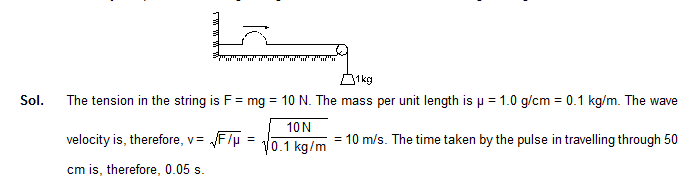4.    The average power transmitted through a given point on a string supporting a sine wave is 0.20 W when the amplitude of the wave is 2.0 mm. What power will be transmiited through this point if the amplitude is increased to 3.0 mm.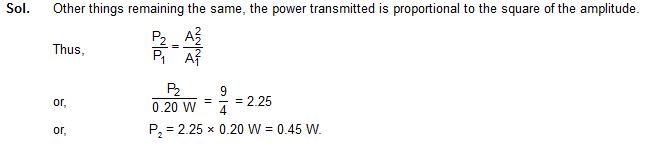5.    Two waves are simultaneously passing through a string. The equations of the waves are given by6.    Two travelling waves of equal amplitudes and equal frequencies move in opposite direction along a string. They interfere to produce a standing wave having the equation.7.    A 50 cm long wire of mass 20 g supports a mass of 1.6 kg as shown in figure. Find the fundamental frequency of the portion of the string between the wall and the pulley. Take g = 10 m/s2.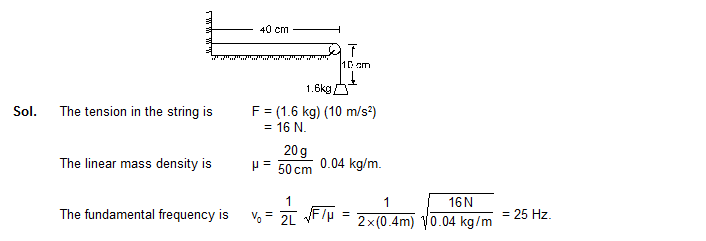8.    In a sonometer experiment, resonance is obtained when the experimental wire has a length of 21 cm between the bridges and the vibrations are excited by a tuning fork of frequency 256 Hz. If  a tuning fork of frequency 384 Hz is used, what should be the length of the experimental wire to get the resonance ?